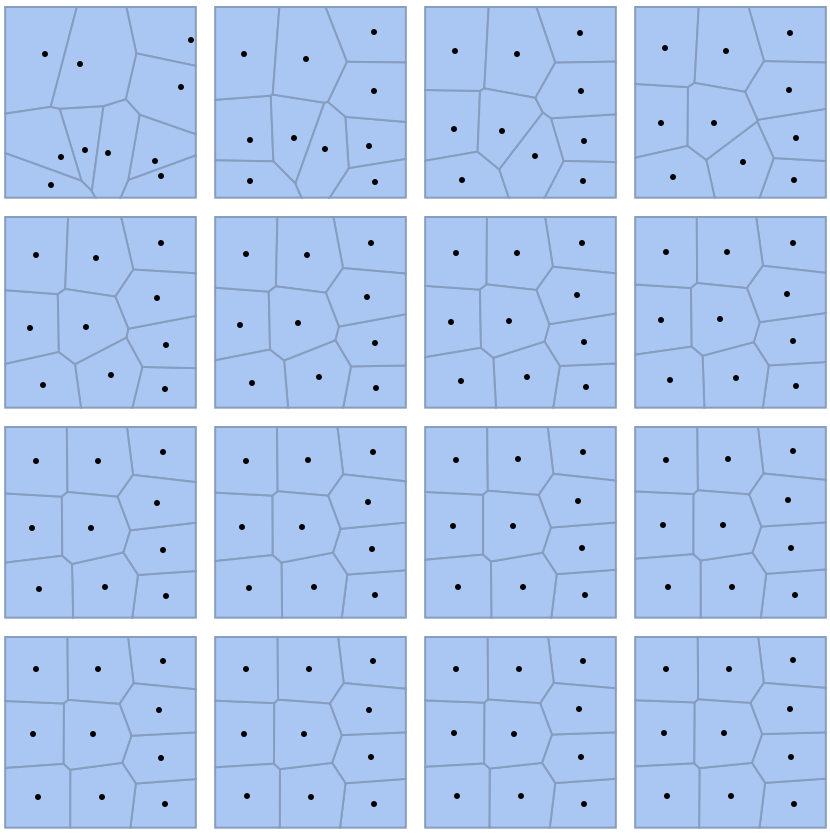#Function Repository Resource:

# LloydAlgorithm

Perform Lloyd's algorithm to find evenly spaced points in a region

Contributed by: Sander Huisman  |  SHuisman
 ResourceFunction["LloydAlgorithm"][pts, {{xmin,xmax},{ymin,ymax}},n] performs n iterations of Lloyd's algorithm in a rectangular region. ResourceFunction["LloydAlgorithm"][pts, reg,n] performs n iterations of Lloyd's algorithm in the region reg.

## Examples

### Basic Examples (2)

Perform five iterations of the algorithm on some random points:

 In:=Out=Spread 15 points evenly in a square box and visualize their neighborhood:

 In:=Out=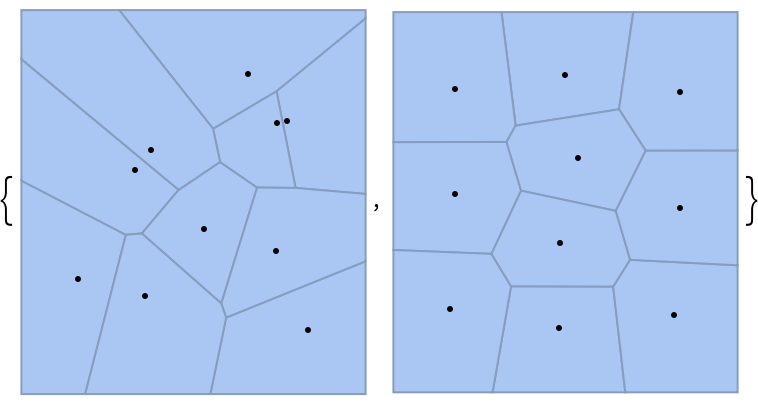### Scope (2)

Perform 15 iterations in a complicated region:

 In:=Out=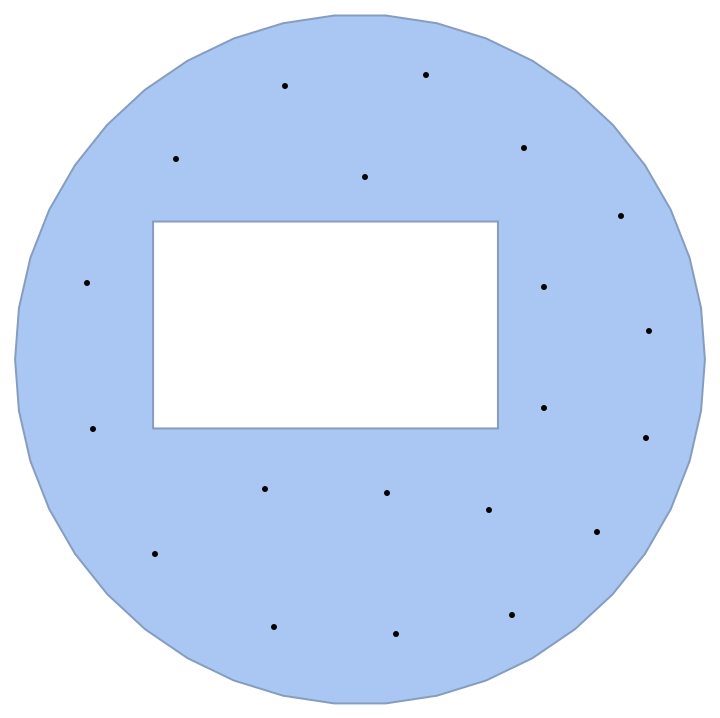Perform 15 iterations in a different complicated region:

 In:=Out=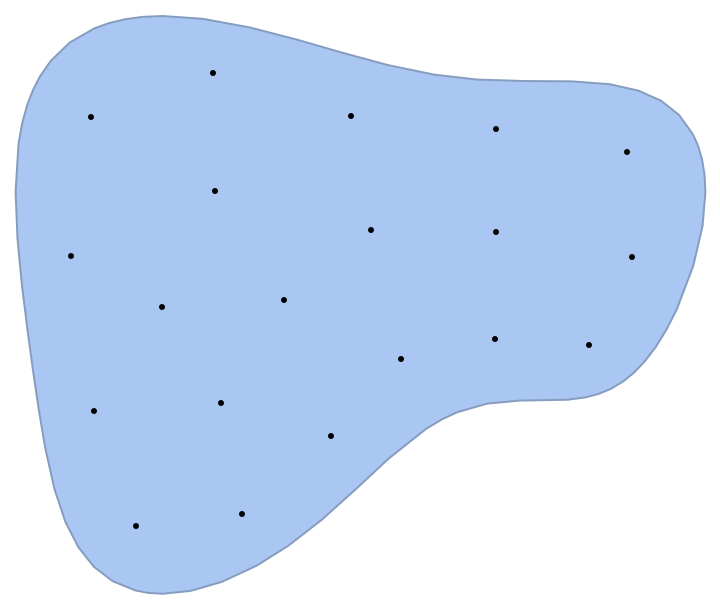### Neat Examples (2)

See how the standard deviation of the Voronoi areas changes with iterations:

 In:=Out=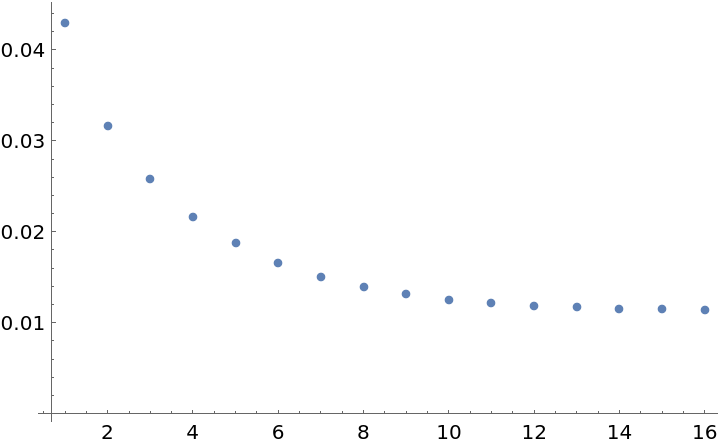Visualize the evolution:

 In:=Out=